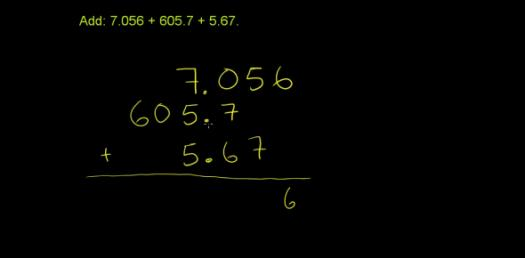6 Questions | Total Attempts: 235SettingsThis basic math Quiz is perfect for helping you when it comes to Adding and Subtracting Decimals. Unlike math problems with whole numbers, one needs to follow specific rules when it comes to solving any math problem involving decimals. This quiz will help you get some much-needed practice in solving these problems, why don’t you give it a try!

• 1.
10.2+3.0=
• A.

14.5

• B.

13.2

• C.

5.2

• D.

None of the above

• 2.
• A.

2.5

• B.

3.0

• C.

2

• D.

None of the above

• 3.
2x5 =
• A.

10

• B.

7

• C.

0

• D.

None of the above

• 4.
Is this statement True or False? Communtive property can be grouped in any way.
• A.

True

• B.

False

• 5.
Is this staement True or False? Associative Property can be grouped any way.
• A.

True

• B.

False

• 6.
What is identity property?
• A.

Numbers can be added in any order.

• B.

Sum of any number is zero.

• C.

Number can be grouped any way.

Related TopicsBack to top